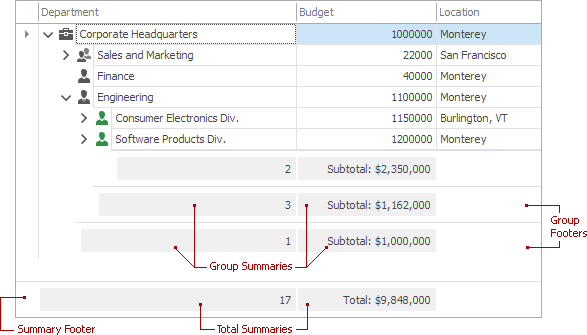# Summary Types

## Summary Types

Summaries allow you to calculate a predefined or custom aggregate function over values of a specific column. The result is displayed under the column for which the summary has been calculated. There are two types of summaries supported by the Tree List control:

• Total Summaries

These are calculated against all nodes, or only root nodes. Total summary values are displayed in the summary footer, at the bottom of the control.

• Group Summaries

These are calculated against each group of nodes. Values of these summaries are displayed in group footers below each group of nodes.The Tree List control supports the following summary functions:

Summary Type Description
Count Calculates the number of rows affected by the summary.
Max Calculates the maximum value among column values affected by the summary.
Min Calculates the minimum value among column values affected by the summary.
Sum Calculates the sum of column values affected by the summary. Applicable only to numeric columns.
Average Calculates the average of column values affected by the summary. Applicable only to numeric columns.